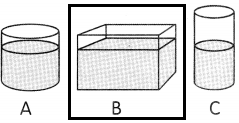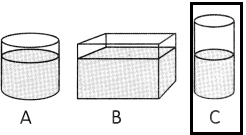# Math in Focus Grade 2 Chapter 9 Practice 1 Answer Key Getting to Know Volume

Practice the problems of Math in Focus Grade 2 Workbook Answer Key Chapter 9 Practice 1 Getting to Know Volume to score better marks in the exam.

## Math in Focus Grade 2 Chapter 9 Practice 1 Answer Key Getting to Know Volume

Fill in the blanks with more, less, or same.

Question 1.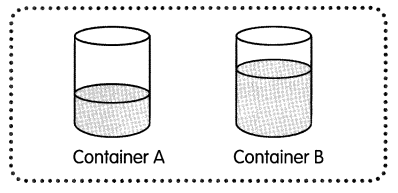Container A and Container B are the same size.

a. Container B has ___ water than Container A.
Explanation:
Observe the picture carefully,
container A is having less water.
container B is having more water.
Therefore, the volume of water in container B is more than in container A.

b. Container A has __ water than Container B.
Observe the picture carefully,
container A is having less water.
container B is having more water.
Therefore, the volume of water in container A is less than in container B.

Question 2.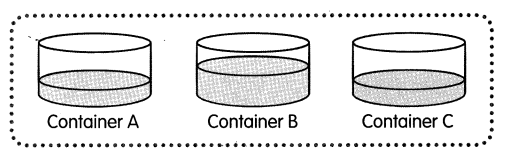Container A, Container B, and Container C are the same size.
a. Container A has ___ water than Container B.
Explanation:
Observe the pictures carefully,
Container A is having less water.
Container B is having more water.
Therefore, the volume of water in container A is less than in container B.

b. Container B has ___ water than Container A.
Explanation:
Observe the pictures carefully,
Container A is having less water.
Container B is having more water.
Therefore, the volume of water in container B is more than in container A.

c. Container A has the ___ amount of water as Container C.
Observe the pictures carefully,
Container A is having the same water.
Container c is having the same water.
Therefore, the volume of water in container A is as same as in container C. Both levels are equal.

Fill in the blanks.

Question 3.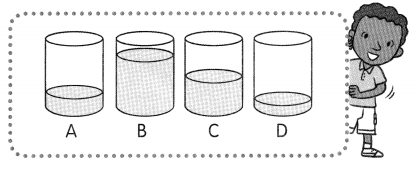The containers are the same size.

a. Container _____ has the greatest amount of water.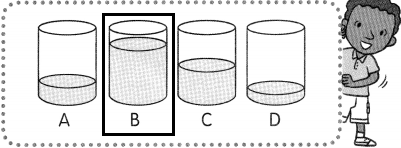The volume of water levels is high in container B.

b. Container ___ has the least amount of water.
The volume of water levels is less in container D.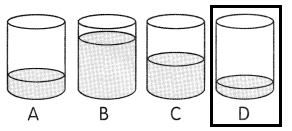Question 4.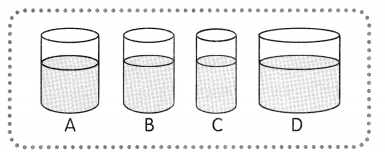a. Container ___ has the greatest amount of water.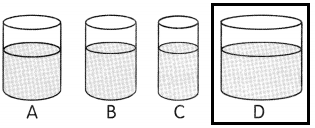Explanation:
We need to determine according to its shape. If the size is more then it can hold a high amount of water.

b. Container ___ has the least amount of water.
In this, the shape is thin compared to other shapes. so it can hold less amount of water.

Question 5.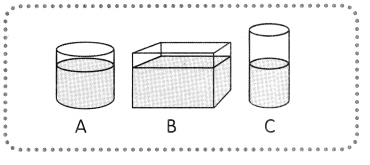a. Container ________ has the greatest amount of water.